xDielectric constantEncyclopedia

The relative permittivity of a material under given conditions reflects the extent to which it concentrates electrostatic lines of flux
Flux
In the various subfields of physics, there exist two common usages of the term flux, both with rigorous mathematical frameworks.* In the study of transport phenomena , flux is defined as flow per unit area, where flow is the movement of some quantity per time...

. In technical terms, it is the ratio of the amount of electrical energy stored in a material by an applied voltage, relative to that stored in a vacuum. Likewise, it is also the ratio of the capacitance of a capacitor using that material as a dielectric, compared to a similar capacitor that has a vacuum as its dielectric.

## Terminology

The relative permittivity of a material for a frequency
Frequency
Frequency is the number of occurrences of a repeating event per unit time. It is also referred to as temporal frequency.The period is the duration of one cycle in a repeating event, so the period is the reciprocal of the frequency...

of zero is known as its static relative permittivity or as its dielectric constant. Other terms used for the zero frequency relative permittivity include relative dielectric constant and static dielectric constant. While they remain very common, these terms are ambiguous and have been deprecated by some standards organizations. The reason for the potential ambiguity is twofold. First, some older authors used "dielectric constant" or "absolute dielectric constant" for the absolute permittivity ε rather than the relative permittivity. Second, while in most modern usage "dielectric constant" refers to a relative permittivity, it may be either the static or the frequency-dependent relative permittivity, depending on context.

Relative permittivity is typically denoted as (sometimes or ) and is defined as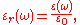where ε(ω) is the complex
Complex number
A complex number is a number consisting of a real part and an imaginary part. Complex numbers extend the idea of the one-dimensional number line to the two-dimensional complex plane by using the number line for the real part and adding a vertical axis to plot the imaginary part...

frequency-dependent absolute permittivity
Permittivity
In electromagnetism, absolute permittivity is the measure of the resistance that is encountered when forming an electric field in a medium. In other words, permittivity is a measure of how an electric field affects, and is affected by, a dielectric medium. The permittivity of a medium describes how...

of the material, and ε0 is the vacuum permittivity.

Relative permittivity is a dimensionless
Dimensionless quantity
In dimensional analysis, a dimensionless quantity or quantity of dimension one is a quantity without an associated physical dimension. It is thus a "pure" number, and as such always has a dimension of 1. Dimensionless quantities are widely used in mathematics, physics, engineering, economics, and...

number that is in general complex
Complex number
A complex number is a number consisting of a real part and an imaginary part. Complex numbers extend the idea of the one-dimensional number line to the two-dimensional complex plane by using the number line for the real part and adding a vertical axis to plot the imaginary part...

. The imaginary portion of the permittivity corresponds to a phase shift of the polarization relative to and leads to the attenuation of electromagnetic waves passing through the medium. By definition, the linear relative permittivity of vacuum is equal to 1, that is ε = ε0, although there are theoretical nonlinear quantum
Quantum electrodynamics
Quantum electrodynamics is the relativistic quantum field theory of electrodynamics. In essence, it describes how light and matter interact and is the first theory where full agreement between quantum mechanics and special relativity is achieved...

effects in vacuum that have been predicted at high field strengths (but not yet observed).

The relative permittivity of a medium is related to its electric susceptibility
Electric susceptibility
In electromagnetism, the electric susceptibility \chi_e is a dimensionless proportionality constant that indicates the degree of polarization of a dielectric material in response to an applied electric field...

, , as .

## Measurement

The relative static permittivity, εr, can be measured for static electric field
Electric field
In physics, an electric field surrounds electrically charged particles and time-varying magnetic fields. The electric field depicts the force exerted on other electrically charged objects by the electrically charged particle the field is surrounding...

s as follows: first the capacitance
Capacitance
In electromagnetism and electronics, capacitance is the ability of a capacitor to store energy in an electric field. Capacitance is also a measure of the amount of electric potential energy stored for a given electric potential. A common form of energy storage device is a parallel-plate capacitor...

of a test capacitor
Capacitor
A capacitor is a passive two-terminal electrical component used to store energy in an electric field. The forms of practical capacitors vary widely, but all contain at least two electrical conductors separated by a dielectric ; for example, one common construction consists of metal foils separated...

, C0, is measured with vacuum between its plates. Then, using the same capacitor and distance between its plates the capacitance Cx with a dielectric
Dielectric
A dielectric is an electrical insulator that can be polarized by an applied electric field. When a dielectric is placed in an electric field, electric charges do not flow through the material, as in a conductor, but only slightly shift from their average equilibrium positions causing dielectric...

between the plates is measured. The relative dielectric constant can be then calculated as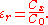For time-variant electromagnetic field
Electromagnetic field
An electromagnetic field is a physical field produced by moving electrically charged objects. It affects the behavior of charged objects in the vicinity of the field. The electromagnetic field extends indefinitely throughout space and describes the electromagnetic interaction...

s, this quantity becomes frequency
Frequency
Frequency is the number of occurrences of a repeating event per unit time. It is also referred to as temporal frequency.The period is the duration of one cycle in a repeating event, so the period is the reciprocal of the frequency...

-dependent and in general is called relative permittivity.

## Practical relevance

The dielectric constant is an essential piece of information when designing capacitor
Capacitor
A capacitor is a passive two-terminal electrical component used to store energy in an electric field. The forms of practical capacitors vary widely, but all contain at least two electrical conductors separated by a dielectric ; for example, one common construction consists of metal foils separated...

s, and in other circumstances where a material might be expected to introduce capacitance
Capacitance
In electromagnetism and electronics, capacitance is the ability of a capacitor to store energy in an electric field. Capacitance is also a measure of the amount of electric potential energy stored for a given electric potential. A common form of energy storage device is a parallel-plate capacitor...

into a circuit. If a material with a high dielectric constant is placed in an electric field
Electric field
In physics, an electric field surrounds electrically charged particles and time-varying magnetic fields. The electric field depicts the force exerted on other electrically charged objects by the electrically charged particle the field is surrounding...

, the magnitude of that field will be measurably reduced within the volume of the dielectric. This fact is commonly used to increase the capacitance of a particular capacitor design. The layers beneath etched conductors in printed circuit boards (PCBs
Printed circuit board
A printed circuit board, or PCB, is used to mechanically support and electrically connect electronic components using conductive pathways, tracks or signal traces etched from copper sheets laminated onto a non-conductive substrate. It is also referred to as printed wiring board or etched wiring...

) also act as dielectrics.

Dielectrics are used in RF
Radio frequency is a rate of oscillation in the range of about 3 kHz to 300 GHz, which corresponds to the frequency of radio waves, and the alternating currents which carry radio signals...

transmission lines. In a coaxial
Coaxial
In geometry, coaxial means that two or more forms share a common axis; it is the three-dimensional linear analogue of concentric.Coaxial cable, as a common example, has a wire conductor in the centre a circumferential outer conductor and an insulating medium called the dielectric separating...

cable, polyethylene
Polyethylene
Polyethylene or polythene is the most widely used plastic, with an annual production of approximately 80 million metric tons...

can be used between the center conductor and outside shield. It can also be placed inside waveguides to form filters. Optical fibers are examples of dielectric waveguide
Waveguide
A waveguide is a structure which guides waves, such as electromagnetic waves or sound waves. There are different types of waveguides for each type of wave...

s. They consist of dielectric materials that are purposely doped with impurities so as to control the precise value of εr within the cross-section. This controls the refractive index of the material and therefore also the optical modes of transmission. However, in these cases it is technically the relative permittivity that matters, as they are not operated in the electrostatic limit.

## Chemical applications

The relative static permittivity of a solvent is a relative measure of its polarity
Chemical polarity
In chemistry, polarity refers to a separation of electric charge leading to a molecule or its chemical groups having an electric dipole or multipole moment. Polar molecules interact through dipole–dipole intermolecular forces and hydrogen bonds. Molecular polarity is dependent on the difference in...

. For example, water (very polar) has a dielectric constant of 80.10 at 20 °C while n-hexane
Hexane
Hexane is a hydrocarbon with the chemical formula C6H14; that is, an alkane with six carbon atoms.The term may refer to any of four other structural isomers with that formula, or to a mixture of them. In the IUPAC nomenclature, however, hexane is the unbranched isomer ; the other four structures...

(very non-polar) has a dielectric constant of 1.89 at 20 °C. This information is of great value when designing separation, sample preparation and chromatography
Chromatography
Chromatography is the collective term for a set of laboratory techniques for the separation of mixtures....

techniques in analytical chemistry
Analytical chemistry
Analytical chemistry is the study of the separation, identification, and quantification of the chemical components of natural and artificial materials. Qualitative analysis gives an indication of the identity of the chemical species in the sample and quantitative analysis determines the amount of...

.

## Complex permittivity

Similar as for absolute permittivity, relative permittivity can be decomposed into real and imaginary parts: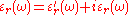.

## Lossy medium

Again, similar as for absolute permittivity, relative permittivity for lossy materials can be formulated as: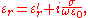in terms of a "dielectric conductivity" σ (units S/m, siemens
Siemens (unit)
The siemens is the SI derived unit of electric conductance and electric admittance. Conductance and admittance are the reciprocals of resistance and impedance respectively, hence one siemens is equal to the reciprocal of one ohm, and is sometimes referred to as the mho. In English, the term...

per meter), which "sums over all the dissipative effects of the material; it may represent an actual [electrical] conductivity caused by migrating charge carriers and it may also refer to an energy loss associated with the dispersion of ε' [the real-valued permittivity]" . Expanding the angular frequency
Angular frequency
In physics, angular frequency ω is a scalar measure of rotation rate. Angular frequency is the magnitude of the vector quantity angular velocity...

ω = 2πc/λ and the electric constant
Electric constant
The physical constant ε0, commonly called the vacuum permittivity, permittivity of free space or electric constant is an ideal, physical constant, which is the value of the absolute dielectric permittivity of classical vacuum...

ε0 = 1/(µ0c2), it reduces to: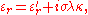where λ is the wavelength, c is the speed of light in vacuum and κ = µ0c/2π ≈ 60.0 S−1 is a newly-introduced constant (units reciprocal of siemens
Siemens (unit)
The siemens is the SI derived unit of electric conductance and electric admittance. Conductance and admittance are the reciprocals of resistance and impedance respectively, hence one siemens is equal to the reciprocal of one ohm, and is sometimes referred to as the mho. In English, the term...

, such that σλκ = εr" remains unitless).

## Metals

Although permittivity is typically associated with dielectric materials, we may still speak of an effective permittivity of a metal, with real relative permittivity equal to one , p. 121). In the low-frequency region (which extends from radiofrequencies to the far infrared region), the plasma frequency of the electron gas is much greater than the electromagnetic propagation frequency, so the complex permittivity ε of a metal is practically a purely imaginary number, expressed in terms of the imaginary unit and a real-valued electrical conductivity -(4.9), p. 122).

• Curie temperature
• Dielectric spectroscopy
Dielectric spectroscopy
Dielectric spectroscopy , and also known as electrochemical impedance spectroscopy, measures the dielectric properties of a medium as a function of frequency...

• Dielectric strength
Dielectric strength
In physics, the term dielectric strength has the following meanings:*Of an insulating material, the maximum electric field strength that it can withstand intrinsically without breaking down, i.e., without experiencing failure of its insulating properties....

• Electret
Electret
Electret is a dielectric material that has a quasi-permanent electric charge or dipole polarisation. An electret generates internal and external electric fields, and is the electrostatic equivalent of a permanent magnet. Oliver Heaviside coined this term in 1885...

• Ferroelectricity
Ferroelectricity
Ferroelectricity is a property of certain materials which possess a spontaneous electric polarization that can be reversed by the application of an external electric field. The term is used in analogy to ferromagnetism, in which a material exhibits a permanent magnetic moment. Ferromagnetism was...

• Green–Kubo relations
• High-k dielectric
High-k Dielectric
The term high-κ dielectric refers to a material with a high dielectric constant κ used in semiconductor manufacturing processes which replaces the silicon dioxide gate dielectric...

• Kramers–Kronig relation
• Linear response function
Linear response function
A linear response function describes the input-output relationship of a signal transducer such as a radio turning electromagnetic waves into music or a neuron turning synaptic input into a response...

• Low-k dielectric
• Loss tangent
Loss tangent
The loss tangent is a parameter of a dielectric material that quantifies its inherent dissipation of electromagnetic energy. The term refers to the tangent of the angle in a complex plane between the resistive component of an electromagnetic field and its reactive component.-Electromagnetic...

• Permittivity
Permittivity
In electromagnetism, absolute permittivity is the measure of the resistance that is encountered when forming an electric field in a medium. In other words, permittivity is a measure of how an electric field affects, and is affected by, a dielectric medium. The permittivity of a medium describes how...

• Refractive index
Refractive index
In optics the refractive index or index of refraction of a substance or medium is a measure of the speed of light in that medium. It is expressed as a ratio of the speed of light in vacuum relative to that in the considered medium....